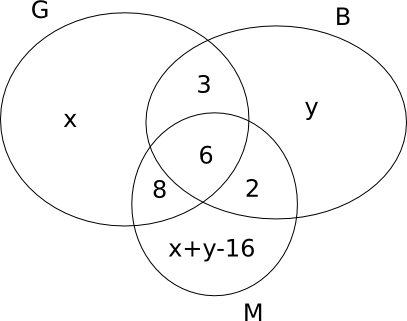### CAT 2003 Question Paper (Leaked) Question 49

Instructions

DIRECTIONS for the following two questions: Answer the questions on the basis of the information given below.

New Age Consultants have three consultants Gyani, Medha and Buddhi. The sum of the number of projects handled by Gyani and Buddhi individually is equal to the number of projects in which Medha is involved. All three consultants are involved together in 6 projects. Gyani works with Medha in 14 projects. Buddhi has 2 projects with Medha but without Gyani, and 3 projects with Gyani but without Medha. The total number of projects for New Age Consultants is one less than twice the number of projects in which more than one consultant is involved.

Question 49

# What is the number of projects in which Medha alone is involved?

SolutionThe total number of projects = 2(3+6+8+2) - 1 = 38 - 1 = 37
So, 19 + 2(x+y) - 16 = 37
=> x+y = 17
The number of projects in which Medha alone is involved is 17-16 = 1
But the number of projects in which Gyani alone is involved cannot be uniquely determined